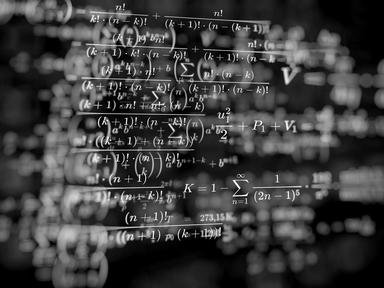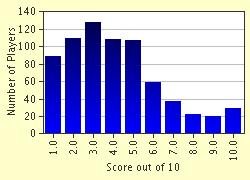FREE! Click here to Join FunTrivia. Thousands of games, quizzes, and lots more!# Calculus Fundamentals Trivia Quiz

### There's a lot more to Calculus than just integrating and differentiating mindlessly. This quiz will cover some of the theorems and concepts behind the area. Some questions are hard, but you shouldn't need to do any actual computations.

A multiple-choice quiz by kevinatilusa. Estimated time: 6 mins.

Author
kevinatilusa
Time
6 mins
Type
Multiple Choice
Quiz #
67,950
Updated
Dec 03 21
# Qns
10
Difficulty
Very Difficult
Avg Score
4 / 10
Plays
3690
Last 3 plays: AdamM7 (6/10), Guest 94 (3/10), Guest 173 (3/10).
- -
Question 1
1. Which of the following can be thought of as a special case of the Mean Value Theorem? Hint

#### NEXT>

Question 2
2. How many REAL roots does the polynomial x to the fifth +12x-7 have? Hint

#### NEXT>

Question 3
3. What is the integral from -Pi over 7 to Pi over 7 of Sin(x cubed)?

#### NEXT>

Question 4
4. What is the integral from 0 to Pi over 2 of (Sine of x divided by the sum of Sine of x and Cosine of x)? Hint

#### NEXT>

Question 5
5. Which of the following statements are true? 1. If a function is Continuous on the closed interval between 0 and 1, it must be Riemann integrable. 2. If a function is Riemann Integrable on that interval, it must be continuous there. 3. If a function is bounded on the closed interval containing 0 and 1 its integral must exist there? Hint

#### NEXT>

Question 6
6. How many roots (real or complex and counting multiple roots the number of times they occur) will a polynomial of degree n have? Hint

#### NEXT>

Question 7
7. Suppose a function satisfies f(0)=1 and f(2)=-1. What can we say about the roots of the function between 0 and 2? Hint

#### NEXT>

Question 8
8. True or False: If two infinitely differentiable functions match in their value and all their derivatives at a point, then the functions must be the same?

#### NEXT>

Question 9
9. Are the following statements true or false: A. If a function is differentiable at 0, it is continuous there. B. If a function is differentiable at 0, its derivative is continuous there? Hint

#### NEXT>

Question 10
10. You have a double integral of a continuous function over a bounded region, and you calculate it by evaluating the integrals one at a time. Whose theorem did you just use?

 (Optional) Create a Free FunTrivia ID to save the points you are about to earn:Select a User ID:Choose a Password:Your Email:

Most Recent Scores
Sep 23 2023 : AdamM7: 6/10
Aug 25 2023 : Guest 94: 3/10
Aug 04 2023 : Guest 173: 3/10

Score DistributionQuiz Answer Key and Fun Facts
1. Which of the following can be thought of as a special case of the Mean Value Theorem?

The mean value theorem states that, if a function is differentiable between the points (a,f(a)) and (b,f(b)) and continuous at the endpoints, then f'(x) must at some x be equal to the slope of the line between the two endpoints. For example, if a car goes 95 miles an hour on average between two cities, it must have been going exactly 95 mph at some point between the cities. Rolle's theorem is the mean value theorem for f(a)=f(b)=0...f' must be 0 somewhere between any two roots of a continuously differentiable function.
2. How many REAL roots does the polynomial x to the fifth +12x-7 have?

This is where Rolle's theorem becomes really useful. We can tell the polynomial has at least one root since it goes to infinity as x does and negative infinity as x becomes very negative, so it has to hit 0 somewhere in between. If it had more than one root, then by Rolle's theorem between the two roots the derivative of the polynomial, 5x to the fourth+12, must be 0.

But 5(x to the fourth)+12 is always positive, so there can't be two roots!
3. What is the integral from -Pi over 7 to Pi over 7 of Sin(x cubed)?

Please say you did NOT try to actually find the antiderivative here! Sin(xcubed)=-Sin((-x)cubed), so the area below the axis to the left of 0 is the same as the area above the axis to the right, and vice versa, so everything cancels leaving 0. On a somewhat related note, if a teacher or professor ever gives you a really really ugly integral, try answering 0. If they ask you how you got it, smile and say 'by symmetry'.

They might be impressed!
4. What is the integral from 0 to Pi over 2 of (Sine of x divided by the sum of Sine of x and Cosine of x)?

Symmetry strikes again! Call the integral I. If we replace x by (Pi over 2)-x, we see that the I is the integral of Cos(x) over ((Sin(x)+Cos(x)). Adding, 2I is the integral of (Cos(x)+Sin(x)) over (Sin(x)+Cos(x))= the integral of 1, which is Pi over 2, so I has value (Pi over 4), or about 0.78. No, I don't know what the antiderivative is, but who needs to find that anyway!
5. Which of the following statements are true? 1. If a function is Continuous on the closed interval between 0 and 1, it must be Riemann integrable. 2. If a function is Riemann Integrable on that interval, it must be continuous there. 3. If a function is bounded on the closed interval containing 0 and 1 its integral must exist there?

1 Is a fundamental theorem about when a function is integrable. 2 is not necessarily true. Say a function was 0 everywhere except at a single point where it was 1. As the rectangle size gets smaller and smaller, the lower sum stays at 0 while the upper sum goes to 0 because the rectangle containing 1 is smaller and smaller. 3 is also sometimes not true, for example if the function was 0 on all the rational numbers and 1 on all the irrational numbers (any rectangle contains a point where f is 0 and a point where it is 1, so the lower integral and the upper integral don't converge to each other).
6. How many roots (real or complex and counting multiple roots the number of times they occur) will a polynomial of degree n have?

This is the fundamental theorem of Algebra. We don't know how many roots are real, but once we include complex roots, we can count them.
7. Suppose a function satisfies f(0)=1 and f(2)=-1. What can we say about the roots of the function between 0 and 2?

It pays to read the fine print on your theorems! The intermediate value theorem only applies for continuous functions, so the function could have any number of roots.
8. True or False: If two infinitely differentiable functions match in their value and all their derivatives at a point, then the functions must be the same?

Surprisingly, no. For example, take the functions f(x)=0 and g(x)= e to the power of (-1 over x squared)(defining g(0)=0). f(0)=g(0)=0 and both functions derivatives of all orders equal to 0! Variations on this function can be used to "glue" together two functions in a way that is infinitely differentiable
9. Are the following statements true or false: A. If a function is differentiable at 0, it is continuous there. B. If a function is differentiable at 0, its derivative is continuous there?

Answer: A is true, but not B

For A: If f was NOT continuous, f(x+h)-f(x) would not approach 0, so the limit defined as the derivative could not exist. Therefore f must be continuous. For B: Take a function g to be 0 at 0 and (x squared times Sine (1 over x)) elsewhere. For x not 0, g' is 2x*Sine(1 over x)+Cos(1 over x), which oscillates wildly around 0. For x equals 0, we can compute the derivative limit directly and find it is 0.

Therefore the derivative exists but is not continuous!
10. You have a double integral of a continuous function over a bounded region, and you calculate it by evaluating the integrals one at a time. Whose theorem did you just use?